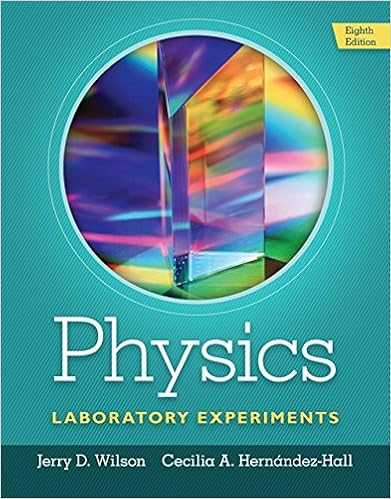# Given by the formula 1 2 2 gm m f d g was measured by

• Notes
• 22
• 67% (3) 2 out of 3 people found this document helpful

This preview shows page 13 - 16 out of 22 pages.

##### We have textbook solutions for you!
The document you are viewing contains questions related to this textbook.The document you are viewing contains questions related to this textbook.
Chapter 11 / Exercise 1
Physics Laboratory Experiments
WilsonExpert Verified
given by the formula 122.Gm mFd=Gwas measured by Cavendish using a torsion balance.G= 6.67 10–11N∙m2/kg2.Earth’s mass (6 1024kg) can be computed from Gand g.Every planet has a different “g.Orbits of planets and comets (and everything else) around the Sun are ellipses.Achieving Earth orbit is like throwing a projectile horizontally at just the right speed (about 7,900 m/s).Here’s an attempt at prioritizing—Law of universal gravitation; mass creates a gravitational field; the field obeys an inverse square law; gravity is one of the four fundamental forces.The gravity force between a pair of objects (weight is an example) is proportional to each mass in the pair; gravity can be measured with a Cavendish torsion balance; it reveals G = 6.67 10–11N∙m2/kg2. Gand gare related; every planet has its own “g.Orbits: satellite orbits imagined in Newton’s ‘cannon’ thought-experiment; orbital velocitiescan be calculated; orbits of planets, comets, etc. are ellipses with the Sun at one focus.Tides: on Earth, primarily due to Moon’s gravity and the fact it is different on the near and far sides of the Earth; neap and spring tides are due to Sun’s added influence.
##### We have textbook solutions for you!
The document you are viewing contains questions related to this textbook.The document you are viewing contains questions related to this textbook.
Chapter 11 / Exercise 1
Physics Laboratory Experiments
WilsonExpert Verified
36CHAPTER2 2.Net forcewhich may beyieldsSimple Harmonic MotionConstantandOpposite to velocityyieldsStraight line motionwithPerpendicular to velocityyieldsParallel to velocityyieldsDecreasing accelerationandTerminal speedDecreasingspeedProportional to and oppositeto displacementCircularmotionProportional to and oppositeto velocityyieldsStraight line motionIncreasingspeedwithANSWERS TO QUESTIONS1.Force is a push or a pull that usually causes distortion and/or acceleration. Examples: weight(force of gravity) pulling down on everything, force of friction between you and your seat, force of friction holding nails and screws in desks, cabinets, walls, etc., and so on.2.Weight is the force of gravity acting on a body. An object is truly weightless only if there is no other body around to exert a gravitational force on it.3.Force of air resistance acting toward the rear of the car and a force of static friction between the car’s roof and the book acting forward.4.Static friction is the type of friction that acts when there is no relative motion between two bodies. Kinetic friction acts when there is relative motion between two bodies in contact. One example of both acting at the same time is a moving car: static friction acts between the
NEWTONSLAWS37road and the tires, and kinetic friction acts between the air and the outside of the car. (See page 49.)5.There are friction forces at both contact surfaces—between the hand and the book on top, and between the book and the table underneath. If the friction force between the hand and the book is larger than that between the table and the book, the book will be dragged along by the hand. In this case static friction acts between the book and the hand, and kinetic friction acts between the table and the book.In the case where the book stays put and the hand slips, it’s very tempting to think that the
•••